This documentation is for scikit-learn version 0.15-gitOther versions

If you use the software, please consider citing scikit-learn.

# 4.5. Pairwise metrics, Affinities and Kernels¶

The sklearn.metrics.pairwise submodule implements utilities to evaluate pairwise distances or affinity of sets of samples.

This module contains both distance metrics and kernels. A brief summary is given on the two here.

Distance metrics are a function d(a, b) such that d(a, b) < d(a, c) if objects a and b are considered “more similar” to objects a and c. Two objects exactly alike would have a distance of zero. One of the most popular examples is Euclidean distance. To be a ‘true’ metric, it must obey the following four conditions:

1. d(a, b) >= 0, for all a and b
2. d(a, b) == 0, if and only if a = b, positive definiteness
3. d(a, b) == d(b, a), symmetry
4. d(a, c) <= d(a, b) + d(b, c), the triangle inequality


Kernels are measures of similarity, i.e. s(a, b) > s(a, c) if objects a and b are considered “more similar” to objects a and c. A kernel must also be positive semi-definite.

There are a number of ways to convert between a distance metric and a similarity measure, such as a kernel. Let D be the distance, and S be the kernel:

1. S = np.exp(-D * gamma), where one heuristic for choosing gamma is 1 / num_features
2. S = 1. / (D / np.max(D))

## 4.5.1. Cosine similarity¶

cosine_similarity computes the L2-normalized dot product of vectors. That is, if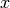and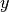are row vectors, their cosine similarity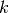is defined as: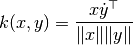This is called cosine similarity, because Euclidean (L2) normalization projects the vectors onto the unit sphere, and their dot product is then the cosine of the angle between the points denoted by the vectors.

This kernel is a popular choice for computing the similarity of documents represented as tf-idf vectors. cosine_similarity accepts scipy.sparse matrices. (Note that the tf-idf functionality in sklearn.feature_extraction.text can produce normalized vectors, in which case cosine_similarity is equivalent to linear_kernel, only slower.)

References:

## 4.5.2. Chi Squared Kernel¶

The chi squared kernel is a very popular choice for training non-linear SVMs in Computer Vision applications. It can be computed using chi2_kernel and then passed to an sklearn.svm.SVC with kernel="precomputed":

>>> from sklearn.svm import SVC
>>> from sklearn.metrics.pairwise import chi2_kernel
>>> X = [[0, 1], [1, 0], [.2, .8], [.7, .3]]
>>> y = [0, 1, 0, 1]
>>> K = chi2_kernel(X, gamma=.5)
>>> K
array([[ 1.        ,  0.36...,  0.89...,  0.58...],
[ 0.36...,  1.        ,  0.51...,  0.83...],
[ 0.89...,  0.51...,  1.        ,  0.77... ],
[ 0.58...,  0.83...,  0.77... ,  1.        ]])

>>> svm = SVC(kernel='precomputed').fit(K, y)
>>> svm.predict(K)
array([0, 1, 0, 1])


It can also be directly used as the kernel argument:

>>> svm = SVC(kernel=chi2_kernel).fit(X, y)
>>> svm.predict(X)
array([0, 1, 0, 1])


The chi squared kernel is given by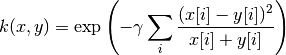The data is assumed to be non-negative, and is often normalized to have an L1-norm of one. The normalization is rationalized with the connection to the chi squared distance, which is a distance between discrete probability distributions.

The chi squared kernel is most commonly used on histograms (bags) of visual words.

References: